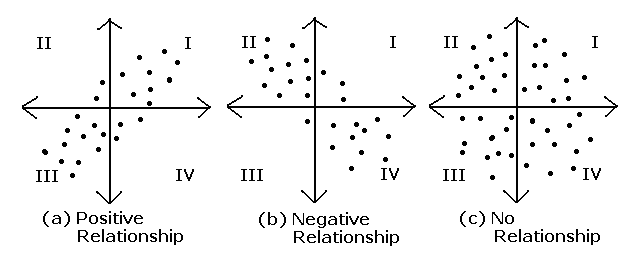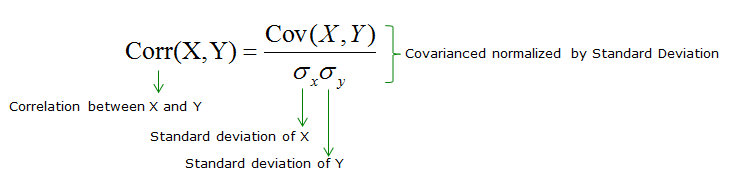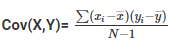# Difference between Covariance and Correlation

Covariance is a measure which shows the extent to which two random variables change in tandem. Correlation gives the indication of how variables are related.In this article we come across the difference between covariance and correlation , definitions and formulas of it.

Covariance and Correlation are two important concepts commonly used in statistics. These topics weigh the linear relationships in the variables. Correlation can be positive, negative and zero. If the correlation is

• positive: an increase in one of the variables results in an increase in the other
• negative: the variables are in opposite directions
• 0: then no relationship existsWhereas, covariance indicates the direction of linear relationships.

## Covariance and Correlation – Definition and Formula

A subset of the population is called a sample. Correlation and covariance are calculated on samples and not populations termed as sample covariance and correlation. Both terms define the relationship and dependency between the variables.

Correlation measures the association between the variables.Covariance explains the joint variability of the variables.Where

xi = data value of x

yi = data value of y

x̄ = mean of x

ȳ = mean of y

N = number of data values.

## Correlation versus Covariance

The function of covariance is correlation. The values of correlation are standardized but covariance values are not. The correlation coefficient can be obtained by dividing the covariance of the variables by the product of their standard deviation values. Standard deviation measures the variability of datasets absolutely. When it is divided by the standard deviation it falls in the range of -1 to +1, which is the range of correlation values. The normalized form of covariance is correlation.

In the formula of covariance, the units are assumed from the product of the units of the variables. Correlation is non-dimensional. It is a measure of the relationship between the variables. The covariance value is affected by the change of scale in the variables. If all the values of one variable are multiplied to a constant and all the values of the other variable are multiplied by a similar or a different constant, the covariance value changes. On doing the same, the correlation value is not affected by the change in scale of the variables.

## Correlation vs Covariance Comparative

 Basis Covariance Correlation Meaning Covariance indicates the extent of the variable being dependent on each other. Higher value denotes higher dependency. Correlation signifies the strength of association between the variables when the other things are constant. Relationship Correlation can be gathered from covariance. Correlation gives the value of covariance on a standard scale. Values Lie between -∞ and +∞ Correlation has limited values in the range of -1 and +1. Scalability Affects covariance Correlation isn’t affected by a change in scale. Units Covariance will have a definite unit as it is concluded from the multiplication of numbers and their units. Correlation is a number without units but includes decimal values.

## Correlation and Covariance For Standardized Attributes

It can be shown that the correlation between attributes is equal to the covariance of two standardized attributes. The first step to this is to standardize the two attributes x and y, obtain their z-scores [x’ and y’] respectively.

$x^{\prime}=\frac{x-\mu_{x}}{\sigma_{x}}, \quad y^{\prime}=\frac{y-\mu_{y}}{\sigma_{y}}\\$

The value of population covariance between the attributes is calculated using the formula,

$\sigma_{x y}=\frac{1}{n} \sum_{i}^{n}\left(x^{(i)}-\mu_{x}\right)\left(y^{(i)}-\mu_{y}\right)\\$

As standardization executes mean-centering, the above equation can be written as

$\sigma_{x y}^{\prime}=\frac{1}{n} \sum_{i}^{n}\left(x^{\prime (i)}-0\right)\left(y^{\prime (i)}-0\right)\\$

If these terms are substituted back using the concepts of standardised attributes, then

\begin{aligned} \frac{1}{n} \sum_{i}^{n}\left(\frac{x-\mu_{x}}{\sigma_{x}}\right)\left(\frac{y-\mu_{y}}{\sigma_{y}}\right) \\ = \frac{1}{n \cdot \sigma_{x} \sigma_{y}} \sum_{i}^{n}\left(x^{(i)}-\mu_{x}\right)\left(y^{(i)}-\mu_{y}\right), \end{aligned}\\

On simplification,

$\sigma_{x y}^{\prime}=\frac{\sigma_{x y}}{\sigma_{x} \sigma_{v}}$

Hence correlation and covariance are the same if the attributes are standardized.

Statistics

Properties of median

## Solved Examples on Covariance And Correlation

Example 1: The coefficient of correlation between x and y is 0.5 and their covariance is 16 and SD of x is 4, then what is the SD of y?

Solution:

Given r = 0.5

Cov (x,y) = 16

σ= 4

σy = cov (x,y) / rσx

= 16 / 0.5 × 4

= 16 / (½) × (42) = 162

= 8

Example 2: If σx = σy and x, y are related by u = x + y; v = x − y, then what is the cov(u,v)?

Solution:

$u=x+y\\v=x-y\\\Rightarrow \bar{u}=\bar{x}+\bar{y}\\\bar{v}=\bar{x}-\bar{y}\\u-\bar{u}=(x-\bar{x})+(y-\bar{y})\\v-\bar{v}=(x-\bar{x})-(y-\bar{y})\\(u-\bar{u})\cdot (v-\bar{v})=(x-\bar{x})^2-(y-\bar{y})^2\\\Rightarrow 1/n \sum (u-\bar{u})(v-\bar{v})=1/n\sum (x-\bar{x})^2-1/x\sum (y-\bar{y})^2\\$

σx2 − σy2 = 0

$\Rightarrow$ cov(u,v)=0

Example 3: What is the correlation between x and a−x?

Solution:

Let u = a − x and therefore

Var (u) = Var (a−x)

=(−1)2 var (x)

= var (x)

= σ2

cov (x, a − x) = cov (x,u)

$r(x,u)=\frac{cov(x,y)}{\sqrt{var(x)var(u)}}$ $=-\frac{\sigma^2}{ \sqrt{\sigma^2,\sigma^2}}$

= -1

Example 3: If the correlation coefficient between x and y is 0.6, covariance is 27 and variance of y is 25, then what is the variance of x?

Solution:

r = 0.6

cov (x, y) = 27

$\sigma^{2}(y)=25 \Rightarrow \sigma(y) = 5\\ r = \frac{\text \ covariance(x, y)}{\sigma(x)\cdot \sigma(y)}\\ \Rightarrow \sigma(x) = \frac{\text \ covariance(x, y)}{r \cdot \sigma(y)} \\ = \frac{27}{\frac{6}{10}*5}\\ =\frac{27*2}{6}=9\\ \sigma^{2}(x)=81\\$

Example 4: If the covariance between x and y is 30, variance of x is 25 and variance of y is 144, then find the correlation coefficient.

Solution:

$cov(x,y)=30 \\ var(x)=25, var(y)=144 \\ r(x,y)=\frac{cov(x,y)}{\sqrt{var(x).var(y)}}\\ r(x,y)=\frac{30}{\sqrt{25*144}}\\ =\frac{30}{5*12}\\ =0.5\\$

Example 5: Let the correlation coefficient between X and Y be 0.6. Random variables Z and W are defined as  Z = X + 5 and W = (Y) / (3). What is the correlation coefficient between Z and W?

Solution:

Given $r_{xy}=0.6\\ z=X+5, w=\frac{Y}{3}\\ b_{zx}=1\Rightarrow b_{wy}=\frac{1}{3}\\ b_{zx} * b_{wy} = 1 * \frac{1}{3}\\ \frac{r_{zw}}{r_{xy}}=\frac{1}{3}\\ \Rightarrow r_{zw}=\frac{r_{xy}}{3}=\frac{0.6}{3}=0.2$